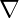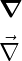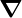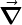# How to denote nabla symbol(∇) or del operator in LaTeX?

Nabla symbol is represented as an inverted triangle(∇). And on the other hand, this nabla symbol is known as a del operator, which you will hear in vector calculus.

In latex, the easiest way to denote a nabla or del operator is to use the \nabla command.

\documentclass{article}
\begin{document}
$\nabla$
\end{document}

Output :Dell operator is a vector operator. Thus, this nabla symbol is represented in the form of bold or with a vector symbol on the nabla symbol.

\documentclass{article}
\begin{document}
$\nabla$
\end{document}

Output :## Use physics package for nabla symbol

Latex has a physics package to represent the operators or symbols of physics. For example

\documentclass{article}
\usepackage{physics}
\begin{document}
$\grad$
\end{document}

Output :physics package automatically converts the nabla symbol to bold. And if you want to add a vector arrow sign to this nabla symbol.

You need to pass the optional argument arrowdel with the physics package.

\documentclass[arrowdel]{article}
\usepackage{physics}
\begin{document}
$\grad$
\end{document}

Output :With this nabla symbol or dell operator, you can identify multiple operators of vector calculus. Such as gradient, curl, laplacian, etc.

However, for these operations, the physics package is used instead of the nabla symbol by default.

And this package contains predefined functions or commands to denote each vector operator.

\documentclass{article}
\usepackage[arrowdel]{physics}
\begin{document}
$\grad{f}$
$\div{\vb{F}}$
$\curl{\vb{F}}$
$\laplacian{f}$
\end{document}

Output :Hopefully, with the help of this tutorial, you have come to understand the use of nabla symbols.

And using pre-defined commands for each physics operator is best practice.#### Md Jidan Mondal

LaTeX expert with over 10 years of experience in document preparation and typesetting. Specializes in creating professional documents, reports, and presentations using LaTeX.